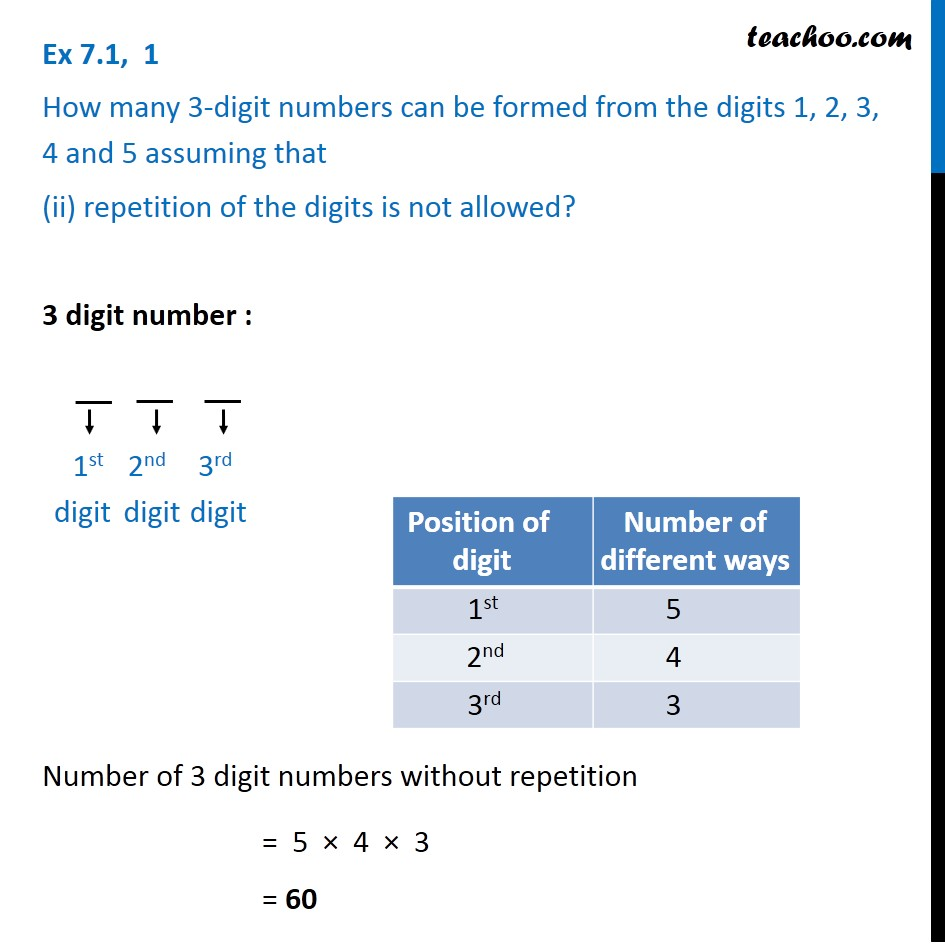1. Chapter 7 Class 11 Permutations and Combinations (Term 2)
2. Serial order wise
3. Ex 7.1

Transcript

Ex 7.1, 1 How many 3-digit numbers can be formed from the digits 1, 2, 3, 4 and 5 assuming that (ii) repetition of the digits is not allowed? 3 digit number : Number of 3 digit numbers without repetition = 5 × 4 × 3 = 60 1st digit 2nd digit 3rd digit